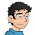## Saturday, January 01, 2011

### Countdown

Just how likely was my pre-midnight countdown? Consider the ordering of 10, 9, 8, 7, 6, 5, 4, 3, 2, 1 and allow the commas to be replaced by one of +, -, *, /, or a concatenation of adjacent numbers (with the added proviso of no concatenation after the 10 to force the generally accepted start of the countdown). We exclude parentheses, so the mathematical statements must be evaluated in the standard order of operations (i.e., multiplications and divisions evaluated before additions and subtractions). Of the 1562500 (4*5^8) possible numerical outcomes, 486501 are unique. Of these, only 51249 are integers (31373 positive), the largest being 10*987654321. The smallest positive integers that do not appear are 1992, 2102, 2133, etc., so every year from 1993 to 2101 will have at least one solution: The largest number of solutions in this interval for a given year is 13 and the average, 616/109. Only two of the solutions start with 10/ (1997 = 10/9*87*6*5 - 43*21 and 2021 = 10/9*8*765/4 + 321). While 1992 is missing from our integer solutions, it may be approximated by the nearby 10 + 9 + 8 + 76*543/21 (as well, if we allow concatenation after the 10, it's 1098 + 765 + 4*32 + 1). You might like to look for one of the four solutions that evaluate to 377/120, the closest that we can get to the irrational number π.

An interesting bonus in last night's countdown exemplar is the symmetry of the operations: +, *, *, /, *, *, +. How common might that be? Out of the 616 solutions in the 1993-2101 interval, I count 12 that qualify:

1994 = 10*9 - 8*7 + 654*3 - 2*1
2001 = 10 - 9*87 + 65*43 - 21
2011 = 10 + 9*8*7/6*5*4 + 321
2020 = 10 - 9 + 8*7 + 654*3 + 2 - 1
2023 = 10*987/6 + 54/3*21
2024 = 10 + 9 + 8*7*6*5 + 4 + 321
2040 = 10 + 9 + 8*7 + 654*3 + 2 + 1
2072 = 10 + 9*8 + 7 + 654*3 + 21
2088 = 10*9*87/6/5/4*32*1
2093 = 10*98 + 7*6*5 + 43*21
2098 = 10 - 98*7 + 65*43 - 21 or 10 - 9*8 + 7 - 6 + 5*432 - 1

1.2.